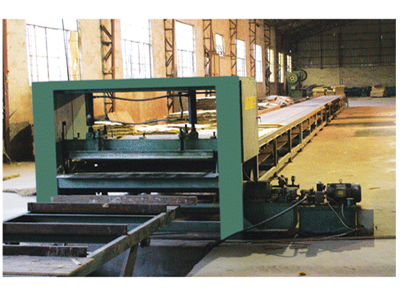•••# 多层板铺板机厂家带来板材常规单位换算

###### 2020-08-31　来自: 多层板铺板机浏览次数:40

（1）1立方米=1000升=1000立方分米=1000000毫升=1000000立方厘米=1000000000立方毫米

（2）1升=1立方分米=1000毫升=1000立方厘米=1000000立方毫米

（3）1立方英尺=1（ft³）=0.0283立方米（m³）=28.317升（liter）=28.317立方分米（dm³）=28317立方厘米=28317000立方毫米

（4）35.315立方英尺(ft)= 6.29桶(bbl)

（5）1千立方英尺(mcf)= 28.317立方米(m³)

（6）1百万立方英尺(MMcf)=2.8317万立方米(m³)

（7）10亿立方英尺(bcf)= 2831.7万立方米(m³)

（8）1万亿立方英尺(tcf)= 283.17亿立方米(m³)

（9）1立方英寸(in)= 16.3871立方厘米(cm³)

（10）1英亩·英尺=1234立方米(m³)

（11）1桶(bbl)= 0.159立方米(m³)= 42美加仑(gal)

（12)1美加仑(gal)= 3.785升（l）

（13）1美夸脱(qt)= 0.946升（l）

（14）1美品脱(pt)= 0.473升（l）

（15）1美吉耳(gi)= 0.118升（l）

（16）1英加仑(gal)= 4.546升（l）### 相关资讯更多>>